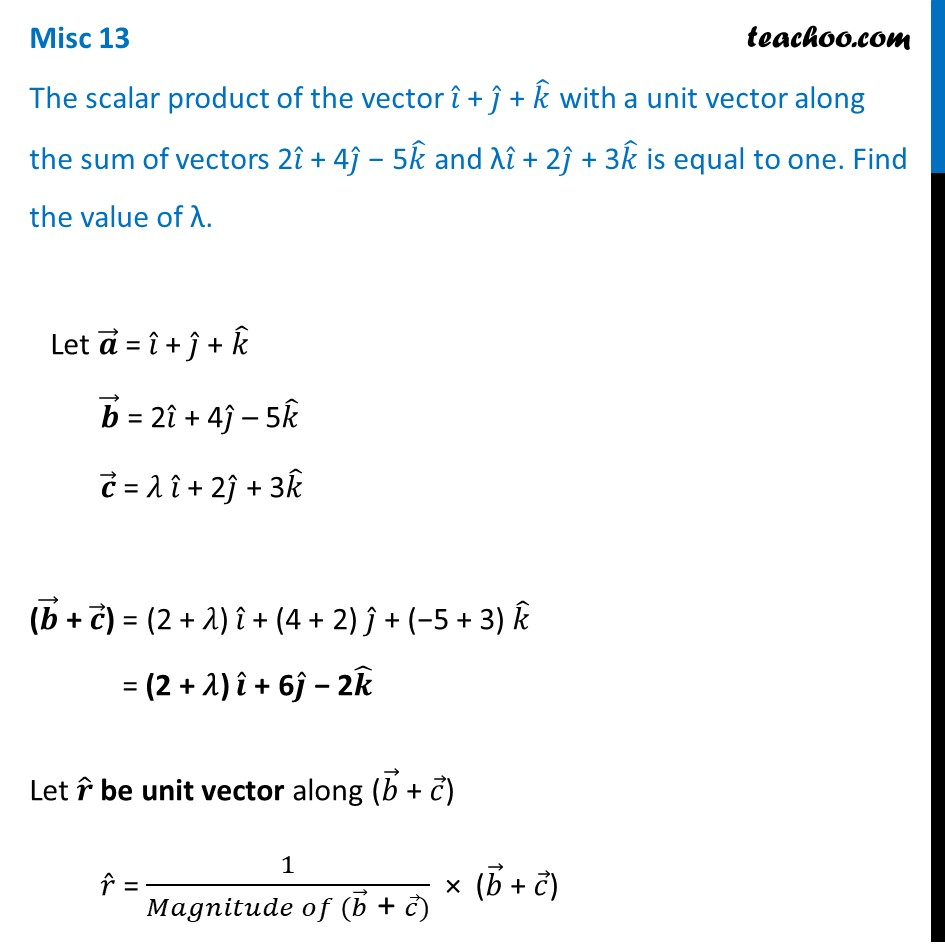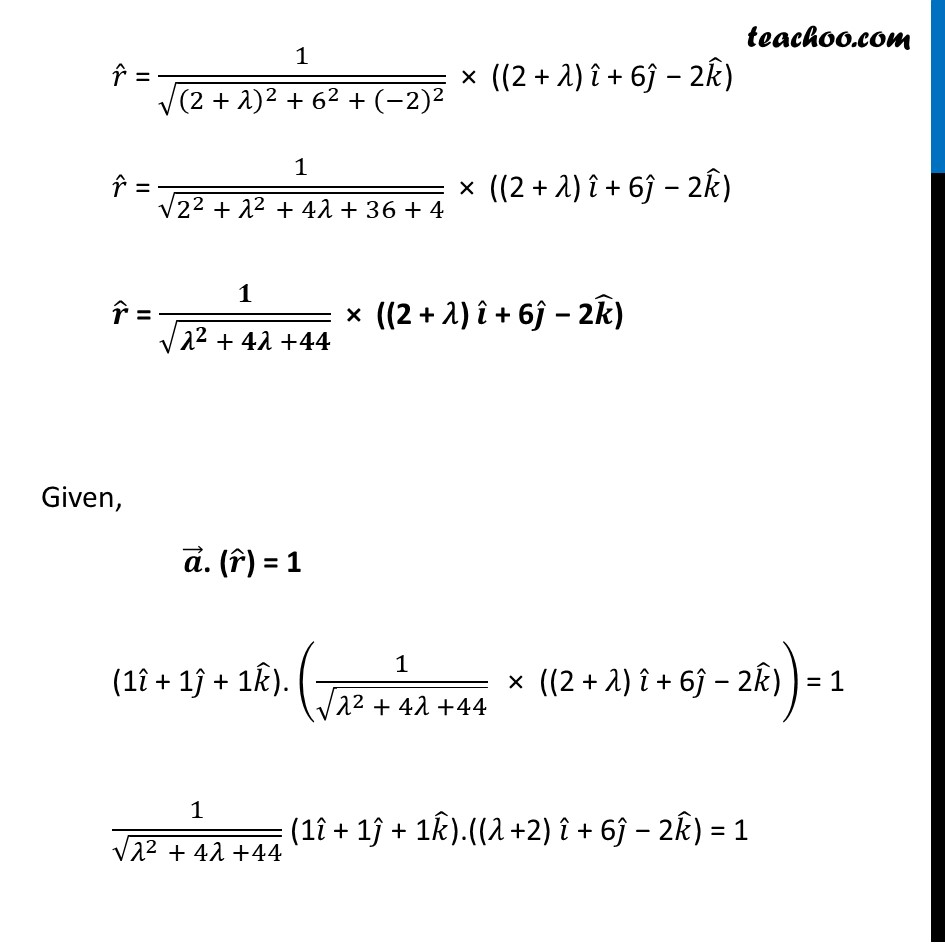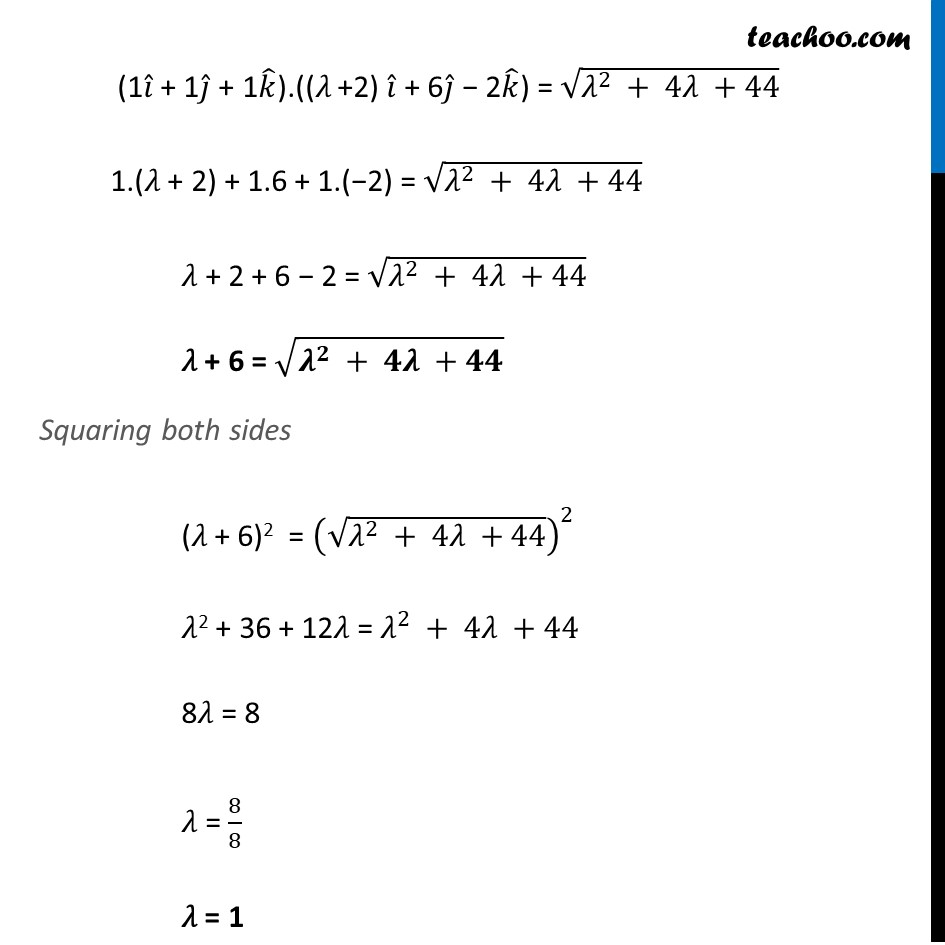Miscellaneous

Chapter 10 Class 12 Vector Algebra
Serial order wiseLearn in your speed, with individual attention - Teachoo Maths 1-on-1 Class

### Transcript

Misc 13 The scalar product of the vector 𝑖 ̂ + 𝑗 ̂ + 𝑘 ̂ with a unit vector along the sum of vectors 2𝑖 ̂ + 4𝑗 ̂ − 5𝑘 ̂ and λ𝑖 ̂ + 2𝑗 ̂ + 3𝑘 ̂ is equal to one. Find the value of λ. Let 𝒂 ⃗ = 𝑖 ̂ + 𝑗 ̂ + 𝑘 ̂ 𝒃 ⃗ = 2𝑖 ̂ + 4𝑗 ̂ – 5𝑘 ̂ 𝒄 ⃗ = 𝜆 𝑖 ̂ + 2𝑗 ̂ + 3𝑘 ̂ (𝒃 ⃗ + 𝒄 ⃗) = (2 + 𝜆) 𝑖 ̂ + (4 + 2) 𝑗 ̂ + (−5 + 3) 𝑘 ̂ = (2 + 𝜆) 𝒊 ̂ + 6𝒋 ̂ − 2𝒌 ̂ Let 𝒓 ̂ be unit vector along (𝑏 ⃗ + 𝑐 ⃗) 𝑟 ̂ = 1/(𝑀𝑎𝑔𝑛𝑖𝑡𝑢𝑑𝑒 𝑜𝑓 (𝑏 ⃗" + " 𝑐 ⃗)) × (𝑏 ⃗ + 𝑐 ⃗) 𝑟 ̂ = 1/√((2 + 𝜆)^2 + 6^2 + (−2)^2 ) × ((2 + 𝜆) 𝑖 ̂ + 6𝑗 ̂ − 2𝑘 ̂) 𝑟 ̂ = 1/√(2^2 + 𝜆^2 + 4𝜆 + 36 + 4) × ((2 + 𝜆) 𝑖 ̂ + 6𝑗 ̂ − 2𝑘 ̂) 𝒓 ̂ = 𝟏/√(𝝀^𝟐 + 𝟒𝝀 +𝟒𝟒) × ((2 + 𝜆) 𝒊 ̂ + 6𝒋 ̂ − 2𝒌 ̂) Given, 𝒂 ⃗. (𝒓 ̂) = 1 (1𝑖 ̂ + 1𝑗 ̂ + 1𝑘 ̂). (1/√(𝜆^2 + 4𝜆 +44) " × ((2 + 𝜆) " 𝑖 ̂" + 6" 𝑗 ̂" − 2" 𝑘 ̂")" ) = 1 1/√(𝜆^2 + 4𝜆 +44) (1𝑖 ̂ + 1𝑗 ̂ + 1𝑘 ̂).((𝜆 +2) 𝑖 ̂ + 6𝑗 ̂ − 2𝑘 ̂) = 1 (1𝑖 ̂ + 1𝑗 ̂ + 1𝑘 ̂).((𝜆 +2) 𝑖 ̂ + 6𝑗 ̂ − 2𝑘 ̂) = √(𝜆^2 + 4𝜆 +44) 1.(𝜆 + 2) + 1.6 + 1.(−2) = √(𝜆^2 + 4𝜆 +44) 𝜆 + 2 + 6 − 2 = √(𝜆^2 + 4𝜆 +44) 𝜆 + 6 = √(𝝀^𝟐 + 𝟒𝝀 +𝟒𝟒) Squaring both sides (𝜆 + 6)2 = (√(𝜆^2 + 4𝜆 +44))^2 𝜆2 + 36 + 12𝜆 = 𝜆^2 + 4𝜆 +44 8𝜆 = 8 𝜆 = 8/8 𝜆 = 1 So, 𝜆 = 1